### Home > CALC > Chapter 5 > Lesson 5.3.1 > Problem5-116

5-116.
1. When interest is compounded quarterly, the annual percentage rate is split into four equal parts and distributed four times during the year, at equally spaced time intervals. Compounding yields more money, because you get interest on previous interest. The easiest way to compute your new amount of money after an interest period is by multiplying the old amount by a suitable number, which we will call the multiplier. What multiplier applies to each of these situations? (Give both the unsimplified form and the simplified form.)

2. Example: 6% annual interest compounded quarterly.

3. Solution: Unsimplified: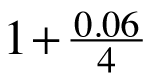. Simplified: 1.015. Homework Help ✎

1. 6% annual interest compounded twice a year.

2. 6% annual interest compounded monthly.

3. 6% annual interest compounded daily.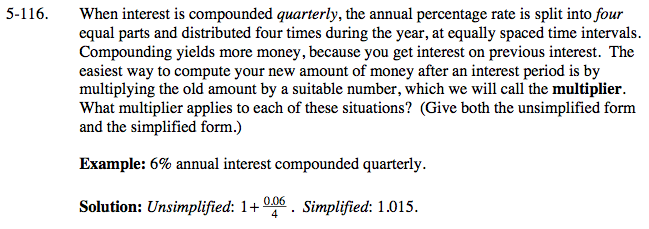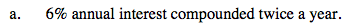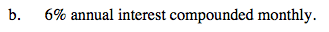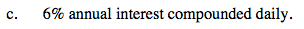Refer to model problems and the example above.